#Quality Digest

Featured Product
This Week in Quality Digest Live
Statistics Features
Donald J. Wheeler
Be careful where you get your information
Steve Moore
You can find quality concepts anywhere
Davis Balestracci
‘...but the problem is that the theory may not make contact with this world’
Jody Muelaner
A primer for quality professionals
Donald J. Wheeler
When should we use the various bias correction factors?
Statistics News
Ability to subscribe with single-user minimum, floating license, and no long-term commitment
A guide for practitioners and managers
Gain visibility into real-time quality data to improve manufacturing process efficiency, quality, and profits
Tool for nonstatisticians automatically generates models that glean insights from complex data sets
Version 3.1 increases flexibility and ease of use with expanded data formatting features
Provides accurate visual representations of the plan-do-study-act cycle
SQCpack and GAGEpack offer a comprehensive approach to improving product quality and consistency
Ask questions, exchange ideas and best practices, share product tips, discuss challenges in quality improvement initiatives
Strategic investment positions EtQ to accelerate innovation efforts and growth strategy
Statistics

## When Are Instruments Equivalent? Part 3

### Comparisons using multiple standards

Published: Monday, June 10, 2019 - 12:03James Beagle IIIDonald J. Wheeler

In Parts One and Two we defined the equivalence of instruments in terms of bias and measurement error based on studies using a single standard. Here we look at comparing instruments for differences in bias or differences in measurement error while using multiple standards.

When we use multiple standards, either known or designated, to compare instruments we can see how the instruments work over a range of values. For our example we shall use three production parts as designated standards (parts A, B, and C). Four inspection fixtures used to measure precision guide rollers will be compared. Fixture 1 was located at the product designer’s plant. Fixtures 2 and 3 were located at the production plants, and fixture 4 was located at the system integrators plant. Due to critical nature of the parameter being measured it was essential that these four fixtures should be equivalent in terms of bias and uncertainty. In this study the same engineer repeatedly measured each of the three parts in the same way using each of the four fixtures. With five measurements of each part on each fixture we end up with 60 measurements. These 60 measurements are organized into 12 subgroups of size five, and limits are computed for each fixture. The resulting average and range charts are shown in figure 1. (The values shown have been coded for simplicity of presentation.)

Figure 2 shows the grand averages, average ranges, and limits for each of these four charts. While we could have used ANOM for the initial analysis of these experimental data, in this case the average and range charts are roughly equivalent. The limits shown in figures 1 and 2 were computed using the standard formulas for average and range charts.

The average and range chart in figure 1 provides us with the ability to quickly understand the issues involved. We can check for internal measurement consistency and perform some simple eye tests for external consistency and bias between instruments.Figure 1: Average and range charts for the four fixturesFigure 2: Chart limits for figure 1

### The “eye tests”

Since no range chart in figure 1 has a point above the upper range limit we may say that each of these fixtures displays internal consistency. However, the differences between the four range charts suggest that these four fixtures may have different levels of measurement error. So we will begin our follow-up analysis by considering if these differences are large enough to justify taking action.

If the instruments are measuring the parts the same way the graphs on the average charts should show reasonable parallelism. Here they do. All four fixtures agree that part A is high, part B is low, and Part C is in the middle. Any failure for the average charts to show the same patterns can be an indication of a serious problem where different instruments measure the same parts in different ways.

At the same time, while we can see that while the four fixtures show the same pattern, they also have different grand averages. These differences suggest possible bias effects between instruments, and later we will evaluate this to see if it is significant enough for action to be taken.

### Checking instruments for differences in measurement error

We will use the analysis of mean ranges (ANOMR) to check for detectable differences in measurement error between the four fixtures. Here we want to compare the m = 4 average ranges. The original study had k = 12 subgroups of size n = 5. The average of all four average ranges will be our central line. This value is known as the overall average range, and in this case it is 2.167. From table 2 at the end of this article, with an overall alpha level of 5 percent, and with n=5, k=12, and m=4, our ANOMR scaling factors are 0.565 and 1.487. These values are multiplied by the overall average range to obtain the limits shown in figure 3.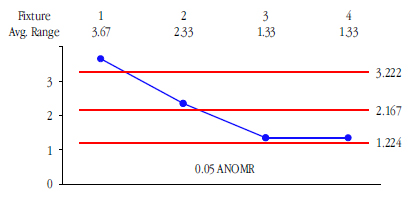Figure 3: ANOMR for comparing average ranges from figure 1

Here we see that the average range for fixture 1 is detectably greater than the overall average range. Thus, we can say that we may have two levels of measurement error present in these data. Now the question becomes one of whether this difference is of any practical importance.

### The probable error of the measurements

One of the most important outputs of any measurement system evaluation is the probable error. Probable error (PE) is the 50th percentile for the distribution of measurement error and represents the effective actual resolution of the measurement system.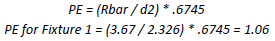This means that half the time a measurement with fixture 1 will err by 1 unit or more, and half the time it will err by one unit or less. Since these measurements are recorded to the nearest whole number of units, they are recorded to the appropriate number of digits, and they are basically good to the last digit.

“Since figure 3 shows the average ranges for fixtures 2, 3, and 4 to be indistinguishable from each other, we combine them when estimating their probable error. The average of these three average ranges is 1.67. Thus we estimate the probable error for fixtures 2, 3, and 4 to be PE = 0.48 units. Since we want the measurement increment to fall somewhere in the range of 0.22 PE to 2.2 PE, and since these fixtures use a measurement increment of 1.0 unit, they also produce values that are good to the last recorded digit. So while fixture 1 displayed more variation than the other fixtures, all of the fixtures produce values that are good to the last recorded digit, and there is no practical difference in measurement resolution between the fixtures.

However, the ANOMR in figure 3 still tells us that fixture 1 has detectably more measurement error than fixtures 2, 3, and 4, and an effort to understand and remove the causes of excessive variation on fixture 1 will likely be necessary.

Using the overall average range of 2.167 we might characterize these four fixtures as producing values with an overall probable error of 0.63 units. This is of importance since the measurement error will help us determine how to react to the suspected bias effects we check for below.”

### Checking instruments for bias effects

We use the analysis of main effects (ANOME) to check for bias effects between the m = 4 fixtures. The original study consisted of k = 12 subgroups of size n = 5. The overall grand average is 62.90. If there is no detectable bias between the four fixtures, their grand averages should all fall within the ANOME limits. The overall average range is 2.167. From table 1 at the end of this article the ANOME scaling factor with a 5-percent overall alpha-level is 0.244. Thus the ANOME detection limits are: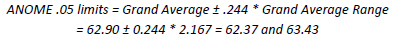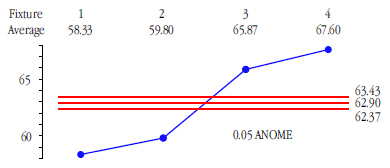Figure 4: ANOME for comparing grand averages from figure 1

Figure 4 shows detectable biases between these four fixtures. By comparing the grand average for each fixture with the overall grand average of 62.90 we estimate these biases as –4.57, –3.10, 2.97, and 4.70 respectively.

In Part One we suggested that biases that are smaller than 1.128 SD(E) or 1.67 PE will not have any impact in practice.

Here our overall estimate of PE is 0.63 units. Thus, biases that are within 1.05 units of each other are unlikely to be important in practice. Since all four of the bias effects estimated above differ by more than 1.05 units, we have to say that all four fixtures are operating at different levels. (Remember, all of these data were obtained by the same engineer, testing the same parts, in the same way, using each of the four fixtures.)Figure 5: Average and range charts for new data following adjustment to remove biases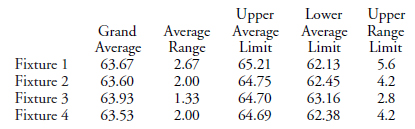Figure 6: Chart limits for figure 5

Here our “eye test” reveals reasonable parallelism between the four average charts, comparable grand averages for each fixture, much greater similarity for the average ranges for the four fixtures and internal consistency for each fixture. We confirm these initial impressions using ANOME and ANOMR charts.

The grand averages for the four fixtures are compared with an ANOME chart. With k = 12, n=5, and m=4, our 5-percent ANOME scaling factor from table 1 is the same as before, 0.244, and our detection limits are: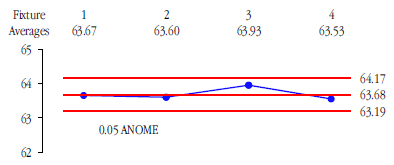Figure 7: ANOME for comparing grand averages from figure 5

With these adjustments to the readings we now have four fixtures with no detectable bias between them. But do the four fixtures have the same amount of measurement error? This question is addressed by the ANOMR chart, which compares average ranges.

As before we use the overall average range to construct the ANOMR chart. The overall average range is 2.00, and the 5-percent ANOMR scaling factors from table 2 are 0.565 and 1.487, resulting in limits of 1.13 and 2.97.Figure 8: ANOMR for comparing average ranges from figure 5

With all of the average ranges within the limits, this ANOMR chart shows no detectable difference in measurement error between the four fixtures.

But what happened to the excessive variation for fixture 1 shown in the initial run? Thankfully, it was easy to keep a record of production data (computer-controlled operation with lot-based data files collected automatically) and it was a simple step to figure out that process variation on fixture 1 appeared to diminish as each “inspection day” progressed. A quick study showed that there was a much longer “warm up” time needed for the laser micrometers used in fixture 1 in comparison to the other fixtures (the method sheets for all four fixtures were revised to reflect this new information).

### Manufacturing specifications

Up to this point, we have avoided any reference to specification limits, but now it is finally necessary. Figure 9 shows an R chart with all confirmation run data factored into the limits.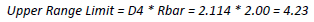Figure 9: Range chart from figure 5 with common limits

With the ranges all falling within the common limits we can now calculate the overall probable error. From figure 9:We can use this value to establish “manufacturing specifications” (see “Where Do Manufacturing Specifications Come From”, by Donald J. Wheeler, Quality Digest Daily, July 2010). 96% manufacturing specifications are found by tightening the watershed specifications by two probable errors. Here the specifications of 70 ± 10 result in watershed specifications of 59.5 and 80.5. Thus we find: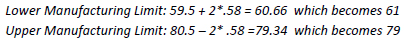When our observed measurement is either 61 or 79, there is at least a 96-percent chance that the product will actually be within the specifications of 60 to 80. (Values between 62 and 78 will be even more likely to be conforming.)

Although these limits appear to be what would be a “good guess” under normal circumstances when setting manufacturing tolerance limits, this process leaves nothing to emotion or subjectivity. Instead, it sets the manufacturing limits based on a statistical foundation derived from measurement capability, the actual behavior of the data, risk assessment, and a balance of tolerance for marginal product combined with an understanding of how that product affects the performance of the system.

### Summary

One of the axioms of data analysis is that we have to detect a difference before we can estimate that difference, and only then can we assess the practical importance of that difference. Since we can detect very small differences when we use enough data, it is crucial that we know how to assess practical importance.

In Part One we demonstrated how measurement error dominates bias effects between instruments when those bias effects are smaller than 1.128 times the standard deviation of measurement error. Here the instruments begin to become equivalent. Depending upon the need for precision in a given application, we may choose to compensate for detectable biases by adjusting the values obtained from each instrument.

In Part Two we introduced the analysis of mean moving ranges (ANOMmR) as a way to test for differences in measurement error between instruments. Here we also further illustrated the adjustment for bias effects between instruments.

In Part Three we have looked at using multiple parts to compare instruments. When we have detectable bias effects, we should always estimate and evaluate the size of these effects relative to measurement error. Based on these three parts, we propose the following guidelines for action:

1. When an instrument fails to display internal consistency it cannot be called a measurement device. When the same thing is measured repeatedly, and ranges for those repeated measurements fall above the upper range limit, we have evidence that the measurement operation is being carried out inconsistently. When this happens the reason for the inconsistency needs to be found and corrected.

2. If two instruments have detectably different levels of measurement error, and if either of the probable errors of these instruments is two or more times the size of the measurement increment, then the two instruments will need to be evaluated separately. As the probable error and measurement increment become similar in size, the measurements begin to be good to the last recorded digit, and measurement error becomes less important in the measurement process.

3. When instruments display equivalent amounts of measurement error, they may be compared for detectable bias effects. When detectable bias effects are smaller than 1.67 probable errors, measurement error will dominate the bias effects. Here the decision regarding the need to make adjustments for the bias effects will depend upon the precision needed in the application where the measurements are being used.

### Tables for ANOM

The following tables are excerpts from more extensive tables given in Analyzing Experimental Data, by Donald J. Wheeler (SPC Press, 2013) and are used with permission.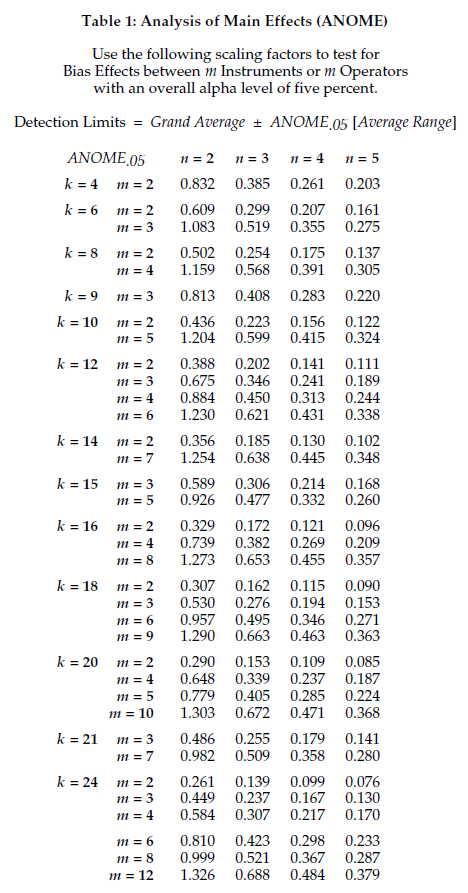### About The Authors### James Beagle III

James Beagle III is a lapsed CQE, CRE, and C6SBB (asking the wrong questions and obsessing about impractical concepts, before realizing what is important) with 35+years experience in process, quality, and reliability engineering. He primarily works with a large manufacturing base supplying data storage component products, where he develops tools for data analysis, designs experiments for product improvements, and works on product and process qualification. In his free time he works on numerical modeling (and is also known to have guitars at home). He can be reached at james@idealstates.net.### Donald J. Wheeler

Dr. Donald J. Wheeler is a Fellow of both the American Statistical Association and the American Society for Quality, and is the recipient of the 2010 Deming Medal. As the author of 25 books and hundreds of articles, he is one of the leading authorities on statistical process control and applied data analysis. Find out more about Dr. Wheeler’s books at www.spcpress.com.

Dr. Wheeler welcomes your questions. You can contact him at djwheeler@spcpress.com